## October 19, 2013

### Murray polygons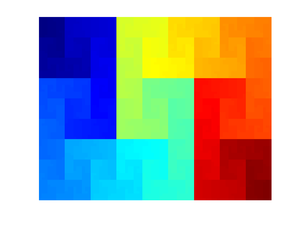Murray polygons are a neat approach to making space-filling curves without recursion, invented by Jack Cole.

Most space-filling curves are made by subdivision: an initial seed curve has segments that are replaced with copies of the seed curve, suitably rotated and scaled. The murray polygon is instead generated incrementally, with each segment added in turn. The trick is that the coordinates of the next point are calculated using "multiple radix arithmetic" (which is how it gets its name, as a loose abbreviation) and Gray codes.

The core idea is to use numbers where each digit has a different radix. Normally each digit has identical radix, whether we write in decimal or binary. But one could for example write numbers so that even digits are in base 10 and odd digits in base 2: 0, 1, 10, 11, 20, 21, 30, 31, 40, 41, 50, 51, 60, 61, 70, 71, 80, 81, 90, 91, 100, 101, 110, 111, 120, ... Addition and subtraction works just like normal, except that one needs to check that when two digits sum to something bigger than the radix for that place there is a carry to the next.

Murray polygons are defined by a series of odd number radices. Even digits denote y-coordinates and odd digits x-coordinates. By selecting different radices one gets different curves.

Just counting upwards doesn't produce a space-filling curve since each digit can only increase or be reset to 0. In order to make a series where digits can also decrease in short steps Gray code is used. A Gray code will only change a single digit at a time as it is incremented. Normally they are binary, but non-binary codes can be constructed, including multiple radix codes. This gives us the pieces we need:

1. Define a multiple radix to use.
2. Set the state to zero.
3. While not done:

1. Increment the state.
2. Calculate its Gray-code.
3. Use the even digits as an x-coordinate and the odd digits as y-coordinate.
4. Convert them to normal numbers and plot.

Why does this work? The Grey code ensures that each increment will only lead to a one unit change in any digit. If it is an even digit the curve moves horizontally, otherwise vertically. The radices determine how many steps will be taken until a carry makes the curve turn (the digit that before the turn was increasing by 1 each step in the Grey coded number will now decrease by 1 each step). And for odd radix sets the end result is a curve that is non-selfintersecting.

Strictly speaking there is a hint of recursion going on here: when calculating the carries in addition, they sometimes cascade. This is what acts as a "hidden" stack.

It is a neat solution to the snake-in-the-box problem.

Here is the simplest murray polygon, the (3)(3) case: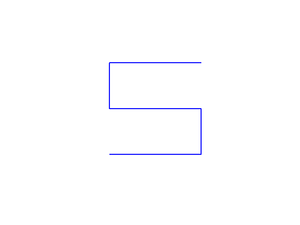followed by the (3)(5):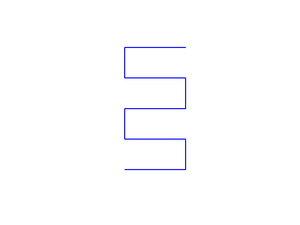The horizontal radix 3 tells us how many steps will be taken before a turn, while 5 tells us how many turns there will be. The case (33)(33) is more complex: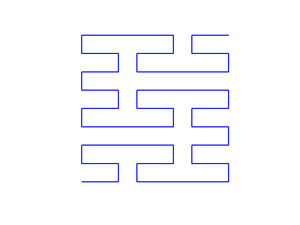Now there are three (3)(3) snakes arranged in the overall shape of a big (3)(3) snake. While this could easily have been done recursively, we did it using an iterative method.

(33)(35) and (33)(53) are similar, but make turns in different places: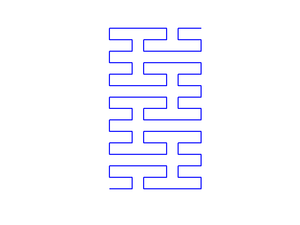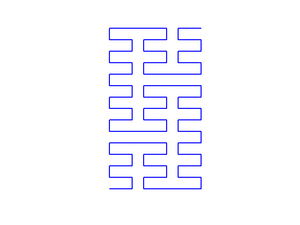(333)(73) shows that there does not have to be the same number of radices for both coordinates (but if you want even more difference you will have to pad with ones):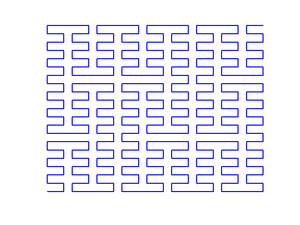Plotting the point order as a function of coordinate in the rectangle gives a 3D image of how the polygon snakes: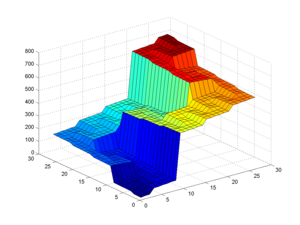For some radices the snaking is pretty minor, while others are more like the twistiness of Hilbert curves.

I generated these curves using a Matlab program derived from Jack Cole's S-Algol program. Despite not knowing any Algol, it was surprisingly easy to do. The algorithm he uses is more clever than the one above, since it avoids having to recalculate Gray codes for every increment. Instead a coded number is maintained and updated only where needed.

My code: murray.m, number_pts.m, change_parities.m and increment.m.

More images on Flickr.

Posted by Anders3 at October 19, 2013 06:01 PM